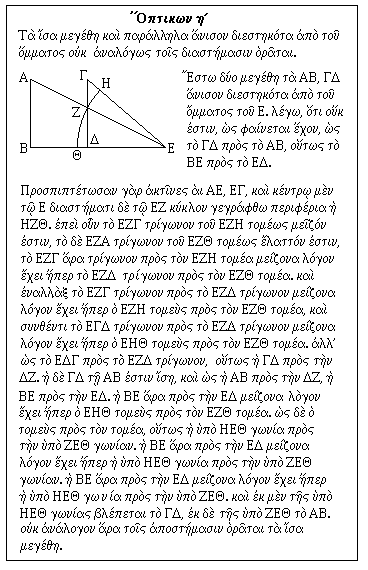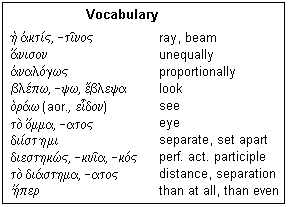## Lesson κδ': A Theorem from the Optics

What seems obvious is not always true

The mathematical theory of perspective was well-developed in ancient times, when it was known as Optics. The theory was lost, then rediscovered in the renaissance by Alberti and others. Euclid's Optics, a treatise on elementary perspective, has survived, and an interesting theorem from it is presented here. The Greek text can be found in the Loeb Classical Library, in Volume I of Selections from Greek Mathematics, pp. 502-505, as well as in Heiberg. There are two recensions of the text, one Theonine and one apparently earlier. This text is from the earlier recension.

Everyone is aware that an object appears smaller and smaller as it moves farther and farther from the eye, and one might well assume that at double the distance, the object appears half the size. If you should ask anyone on the street, this will probably be their opinion. Now this is approximately true, very closely so for distant objects, but it is not exactly true for nearby objects. Eli Maor mentions this in Chapters 2 and 12 of Trigonometric Delights (Princeton, NJ: Princeton University Press, 1938; ISBN 0-691-05754-0), but does not give the theorem or its proof. The theorem is Proposition 8 of Euclid's Optics, and it is an interesting and pretty theorem.The proposition is shown at the right, and a Vocabulary is provided below. The statement of the proposition is: "The equal and parallel magnitudes unequally distant from the eye are not seen proportionally to the distances." The fundamental reason for this is that the arcs such as ZΘ that subtend the visual angle are longer than the perpendiculars such as ZΔ, or that the sine or tangent of an angle is less than the angle. Euclid now uses the fact that the areas of triangles on the same base are proportional to their altitudes, and the areas of sectors of the same radius are proportional to their angles. This saves him from having to consider the length of a curved line. We would be comfortable enough with this, but he was not.

To proceed: "Let the two magnitudes AB, ΓΔ [be] unequally distant from the eye E. I say, that it is not, as it would seem, that ΓΔ is to AB, as BE is to EΔ. Let the rays AE, EΓ be projected, and let be drawn the arc HZΘ with the center E and radius [distance] EZ. Therefore, since the triangle EZΓ is greater than the sector EZH, and the EZΔ triangle is less than the sector EZΘ, therefore the EZΓ triangle has a greater ratio to the EZH sector than even the EZΔ triangle to the EZΘ sector. And invertendo the EZ&GAmma; triangle has a greater ratio to the EZΔ triangle than even the EZH sector to the EZΘ sector, and componendo, the EΓΔ triangle has a greater ratio to the EZΔ triangle than even the EHΘ sector to the EZΘ sector. But as the EΔΓ [is] to the EZΔ triangle, so [is] ΓΔ to ΔZ. And ΓΔ is equal to AB, and as AB is to ΔZ, BE [is] to EΔ. Therefore, BE has a greater ratio to EΔ than even the EHΘ sector to the EZΘ sector. And as the sector is to the sector, , so the angle HEΘ is to the angle ZEΘ.Therefore, BE has a greater ratio to EΔ than even the angle HEΘ to the [angle] ZEΘ. And ΓΔ is seen under the angle HEΘ and AB under ZEΘ. Therefore, the equal magnitudes are not seen proportionally to the distances." Is that clear?

In the proof, the manipulations of the ratios were presented in their original form, which cannot be directly understood unless you are familiar with the terms. Algebra makes it all very clear, and I encourage you to go back to the proof and write it algebraically to see what is happening. The first manipulation, invertendo, is this: if a/b > c/d, then ad > bc, multiplying both sides by bd. Now, dividing by cd, we find a/c > b/d. Hence, the two middle terms (b,c) can be interchanged without altering the inequality. The second manipulation, componendo, is this: adding unity to both sides, we find a/b + 1 > c/d + 1. Multiplying by bd, we get ad + bd > cb + bd, or (a+b)d > (c+d)b. Dividing by bd, we get (a+b)/b > (c+d)/d. These transformations also apply to equalities, of course, as well as to inequalities. Without algebra, they become rules to be followed instead of manipulations according to the general rules of algebra.

If the magnitudes AB and ΓΔ are taken as unity, then the distances BE and ΔE are the cotangents of the angles subtended. We can turn the theorem into a trigonometric inequality, that if β > α, then tan β / tan α > β / α. It is easy to verify this for a few angles if you do not believe it. As the angles become small, the tangents approach the values of the angles in radian measure, and now the angles subtended are inversely proportional to the distances.The first word in the vocabulary is the source of the English word "actinic" for radioactive. The commonest interpretation of light and vision in classical times was in terms of straight rays from the eye and from sources of light. A ray from the sun would illuminate an object, and make it visible when touched by a ray from the eye. Epicureans, however, held that light was a subtle substance emitted from sources of light that was reflected by an object to enter the eye, producing the sensation of vision. The latter view is, of course, closer to the modern one, but there was then no way to verify one view or the other, so the simpler, and wrong, one was chosen. To all considerations of perspective, reflection and refraction it makes no difference whatsoever. All rays of light are reversible, and take place the same way in both directions, in media that are stationary or moving with small velocities. Can you prove that light travels from an object to the eye rather than from the eye to an object? Finding the truth here is a little more difficult than it would appear on the surface. Most people these days repeat scientific facts with absolutely no conception of how to show that they are true, which is not much better than not knowing them at all.

a)ni/son and a)nalo/gws are adverbs, the first an accusative, and the second formed in the "regular" way from an adjective. The middle voice of ble/pw means to "seem" or "appear." The verb o/)raw undergoes stem changes in its conjugation, with various alternatives. From the aorist, we get the word "eidetic." The endings of this verb contract with the accented α. dii/sthmi is an important verb with many derivatives, several of which are used here. We are already familiar with h), soft breathing, as "than" in comparisons. The suffix per intensifies the contrast, which we can approximate by saying "than even."

This theorem is interesting in that it proves that a reasonable and simple supposition is, in fact, wrong, and that matters are more complicated than might be supposed. It is an example that mere thought is no reliable way to the truth, only rigorous proof. In today's world as in Euclid's, many things are believed true because they seem obvious and reasonable, feel right, and are widely accepted, but which are, in fact, false.